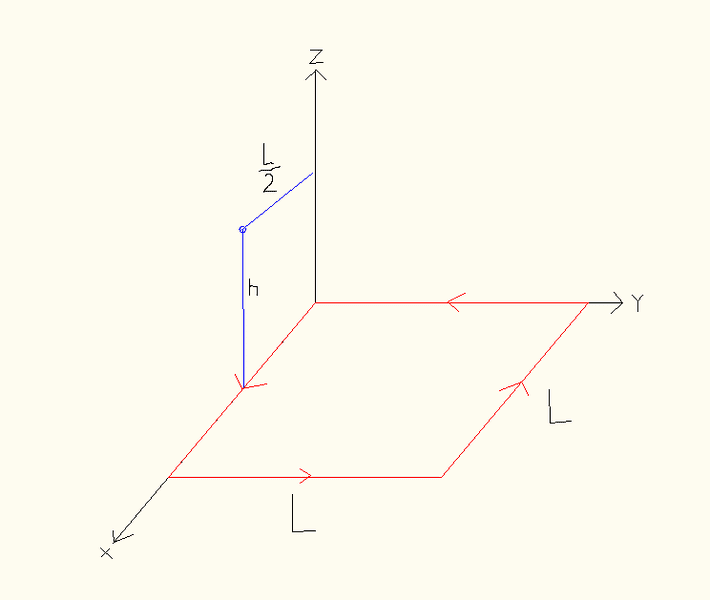# Mag. field of square loop at far away point

• vwishndaetr

#### vwishndaetrI had to find the magnetic field due to each side at point P, a distance h above x-axis and L/2 from z-axis.

In previous parts, summing up all the sides, I came up with a net magnetic field at point P, of:

$$B_{net}=\frac{\mu_0I}{4\pi}\left[\left(\frac{L}{h\sqrt{h^2+\frac{L^2}{4}}}+\frac{L^2}{(h^2+L^2)\sqrt{h^2+\frac{5L^2}{4}}}\right)\hat{y}+\left(\frac{2Lh}{(h^2+\frac{L^2}{4})\sqrt{h^2+\frac{5L^2}{4}}}+\frac{Lh}{(h^2+L^2)\sqrt{h^2+\frac{5L^2}{4}}}\right)\hat{z}\right]$$

The final part of the problem asks to find the magnetic field at a far away point P(L/2,y0,z0) where y0 and z0 are much larger than L.

How do I tackle this? I know I am not going to have to redo the entire calculation for a different point. Is it safe to just take the limit,

$$\lim_{h\rightarrow \infty} B_{net}$$

The way I look at it, my equation is for the point P(L/2,0,h). For the far away point, my x isn't changing, but y and z are. So by taking this limit, I get my z very far, but how to do it for a far y as well?

Am I thinking this over right?

Am I thinking this over right?
I think you are over-thinking it. Very far from the xy plane the loop looks like a magnetic dipole and I believe you are expected to treat it as such.

Ok. Thats a step.

So can I use my current result and alter it to accommodate for such change, or do I have to redo the derivation?

Thanks.

Ok I got it. Following through with what was mentioned above, I arrived with a new formula, but for a dipole as mentioned. All is clear, thank you. :)